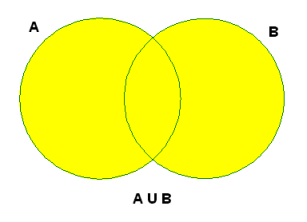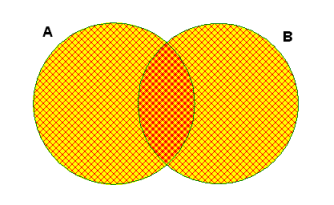#Advanced Set Concepts

Need to review basic properties of sets?  Introduction to Sets

Need to review interval and list notation for sets?  Interval and List Notation

## Set-Builder Notation

Often, it is convenient to describe a set by stating a property that members of the set must satisfy. In such cases, set-builder notation comes to the rescue.

Set-builder notation always has the following general form:

$$\cssId{s9}{\{} \cssId{s10}{x} \cssId{s11}{\ |\ } \cssId{s12}{\text{ a property that }\ x\ \text{ must satisfy }} \cssId{s13}{\}}$$

The vertical bar,   ‘$\,|\,$’,   is read as such that or with the property that.

For example:

$\{ x\ |\ x\gt 0\}$
‘the set of all $\,x\,$ with the property that $\,x\,$ is greater than zero’

Or,

$\{ x\ |\ x\gt 0\}$
‘the set of all $\,x\,$ such that $\,x\,$ is greater than zero’

Here's how those words attach themselves to different parts of the sentence:

$$\cssId{s24}{\overset{\text{the set of all}}{\overbrace{\strut\ \ \{\ \ }}} \cssId{s25}{\overset{\text{ex}}{\overbrace{\strut\ \ x\ \ }}} \cssId{s26}{\underset{\text{with the property that}}{\underbrace{ \overset{\text{such that}}{\overbrace{\strut\ \ |\ \ }}}}} \cssId{s27}{\overset{\text{ex}}{\overbrace{\strut\ \ \ x\ \ }}} \cssId{s28}{\overset{\text{is greater than}}{\overbrace{\strut\ \ \gt\ \ }}}\ \ \cssId{s29}{\overset{\text{zero}}{\overbrace{\strut\ \ 0\ \ }}}\ \ \}$$

Notice that the closing brace,   ‘$\,\}\,$’,   is not verbalized. It is required, however, since it marks the end of the set structure.

Of course, sets often have different names. In the example above, the set could alternatively be described using interval notation: $$\cssId{s34}{\{ x\ |\ x\gt 0 \}} \cssId{s35}{=} \cssId{s36}{(0,\infty )}$$

Set-builder notation uses the concept of dummy variable. Roughly, a dummy variable is just a name given to something so that we have a way to talk about it; the name used doesn't affect the result.

Dummy variables are used in function notation: ‘$\,f(x)= x+2\,$’ describes the rule ‘take a number and add two’.  Here, the dummy variable is $\,x\,.$  ‘$\,f(t)=t+2\,$’ also describes the rule ‘take a number and add two’.  Here, the dummy variable is $\,t\,.$

The set $\,\{x\ |\ x\gt 0\}\,$ can be written, using the dummy variable $\,t\,,$ as $\{t\ |\ t\gt 0\}\,.$ Or, it can be written, using the dummy variable $\,w\,,$ as $\,\{w\ |\ w\gt 0\}\,.$ Of course, it's a good idea to stick to the normal conventions for naming variables: for real numbers, use letters near the end of the alphabet; for integers, use letters near the middle of the alphabet.

## Connectives for Sets

Addition, ‘$\,+\,$’, is a connective for numbers. That is, two numbers $\,x\,$ and $\,y\,$ can be connected to get a new number, $\,x+y\,.$

Similarly, ‘$\,\cup\,$’ (union) and ‘$\,\cap\,$’ (intersection) are connectives for sets; they combine two sets to give a new set.

### Set Union

Let $\,A\,$ and $\,B\,$ be sets. The set ‘$\,A\cup B\,$’ is read as ‘$\,A\,$ union $\,B\,$’. By definition: $$\cssId{s76}{A\cup B} \cssId{s77}{=} \cssId{s78}{\{ x\ |\ x\in A \ \text{ or }\ x\in B\}}$$ (This is the mathematical word ‘or’ being used here.) Thus, to find $\,A\cup B\,,$ you put in everything from $\,A\,,$ and also everything from $\,B\,.$

Venn diagrams are illustrations that are useful for showing the relationship between sets. In the Venn diagram below, $\,A\,$ is the left circle, $\,B\,$ is the right circle, and $\,A\cup B\,$ is shaded.### Set Intersection

The set ‘$\,A\cap B\,$’ is read as ‘$\,A\,$ intersect $\,B\,$’. By definition: $$\cssId{s94}{A\cap B} \cssId{s95}{=} \cssId{s96}{\{ x\ |\ x\in A \ \text{ and }\ x\in B\}}$$ (This is the mathematical word ‘and’ being used here.) Thus, $\,A\cap B\,$ consists of everything that is common to both $\,A\,$ and $\,B\,$;   i.e., the overlap of $\,A\,$ and $\,B\,.$

In the Venn diagram below, the double-hatched (darker) area in the middle is $\,A\cap B\,.$### Set Complement

Let $\,U\,$ be a set (the universal set), and let $\,A\,$ be a subset of $\,U\,.$ The set $\overline{A}\,$ is read as ‘$\,A\,$ bar’ or ‘the complement of $\,A\,$’ and is defined by: $$\cssId{s111}{\overline{A}} \cssId{s112}{=} \cssId{s113}{\{ x\ |\ x\in U \ \text{ and }\ x\notin A\}}$$ Thus, $\,\overline{A}\,$ is everything that is not in $\,A\,$;   it is everything that is outside of $\,A\,.$

In English, the word complement means to fill out or to make a whole, and the set $\,\overline{A}\,$ ‘fills out’ the set $\,A\,$ to give all of $\,U\,.$

In the Venn diagram below, the universal set $\,U\,$ is the rectangle, the subset $\,A\,$ is the circle, and $\,\overline{A}\,$ is the yellow region inside the rectangle, but outside the circle.## Examples

Let $\,A=\{1,2,3\}\,$ and $\,B=\{3,4,5,6\}\,.$

Then, $\,A\cup B = \{1,2,3,4,5,6\}\,.$ Throw in everything from $\,A\,.$ Throw in everything from $\,B\,.$ If something happens to be in both sets, just throw it in once.

Also, $\,A\cap B = \{3\}\,.$ For finite sets, it's most efficient to start with the set that has the fewest members (if there is one). In this case, $\,A\,$ has three members and $\,B\,$ has four members, so start with $\,A\,.$ Go through each member of $\,A\,,$ asking:

‘Is it in the other set?’

If the answer is yes, then throw that member in the intersection. In this example, there's only one member that is in both sets.

If $\,U=\{1,2,3,4,5,6\}\,,$ then $\,\overline{A} = \{4,5,6\}\,.$

If $\,U\,$ is the interval $\,[1,3]\,,$ then $\,\overline{A} = (1,2)\cup (2,3)\,.$

Notice that $\,\overline{A}\,$ depends on the universal set!